Topics: AI | Data Science | Data Visualization | Deep Learning | Machine Learning | NLP | Python | R | Statistics

KDnuggets Home » News » 2016 » Nov » Tutorials, Overviews » How Bayesian Inference Works ( 16:n41 )

#How Bayesian Inference Works

http likes 324

Bayesian inference isn’t magic or mystical; the concepts behind it are completely accessible. In brief, Bayesian inference lets you draw stronger conclusions from your data by folding in what you already know about the answer. Read an in-depth overview here.### Bayesian inference at the veterinarian

My dog is named Reign of Terror. When we go to the vet, she squirms on the scale. That makes it hard to get an accurate reading. Getting an accurate weight is important, because if her weight has gone up, we have to reduce her food intake. She loves her food more than life itself, so the stakes are high.

On our last visit, we got three measurements before she became unmanageable: 13.9 lb, 17.5 lb and 14.1 lb. There is a standard statistical interpretation for this. We can calculate the mean, standard deviation and standard error for this set of numbers and create a distribution for Reign’s actual weight.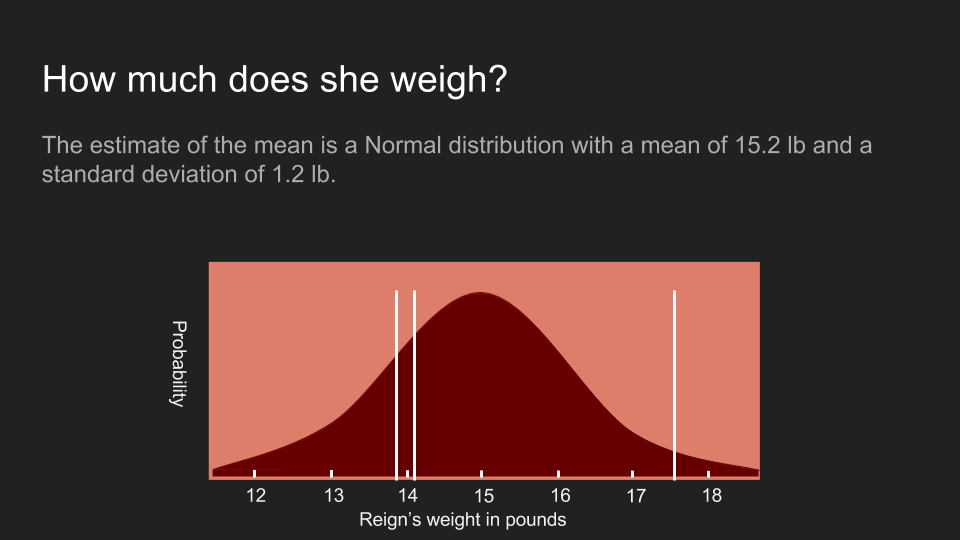This distribution shows what we believe about her weight using this approach. It is normally distributed with a mean of 15.2 pounds and standard error of 1.2 pounds. The actual measurements are shown as white lines. Unfortunately this curve is unsatisfyingly wide. While at the peak is at 15.2 pounds, the probability distribution shows that it could easily be as low as 13 pounds or as high as 17 pounds. It's much too wide a range to make any kind of confident decision. When confronted with results like this, it is common to return and gather more data, but in some cases this is not feasible or is too expensive. In our case, Reign’s patience had been used up. We’re stuck with the measurements we already have.

This is where Bayes’ Theorem comes in. It is useful in making the most out of small data sets. Before we apply it, it's useful to revisit the equation and look at the various terms.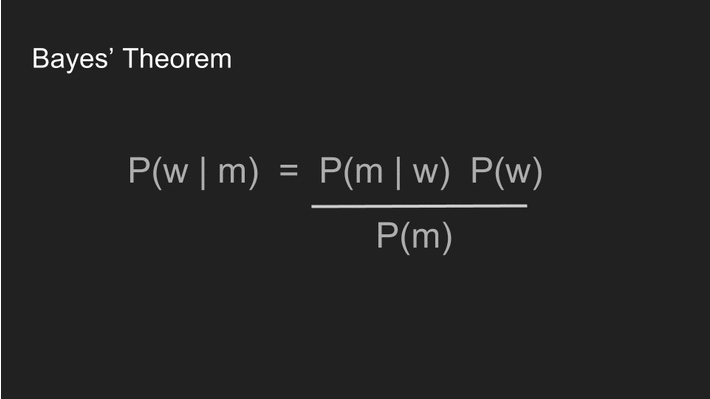We substitute “w” (weight) and “m” (measurements) for “A” and “B” to make it clear how we’re going to use it. The four terms each represent a different part of the process.

The prior, P(w), shows our prior beliefs. In this case, it shows what we believe about Reign’s weight before we even put her on the scale.

The likelihood, P(m | w), shows the probability that our measurements would occur for a particular weight. It’s also called the likelihood of the data.

The posterior, P(w | m), shows the probability of Reign being a given weight, given the measurements we made. This is what we are most interested in.

Probability of data, P(m), shows the probability that any given data point will be measured. For now we’ll assume this is a constant, that is, that the scale is unbiased.

It's not a terrible idea to start by being perfectly agnostic and making no assumptions about the result. In this case, we assume that Reign’s weight is equally likely to be 13 pounds or 15 pounds or 1 pound or 1,000,000 pounds and let the data speak. To do this, we assume a uniform prior, meaning that its probability distribution is a constant for all values. This lets us reduce Bayes’ Theorem to P(w | m) = P(m | w).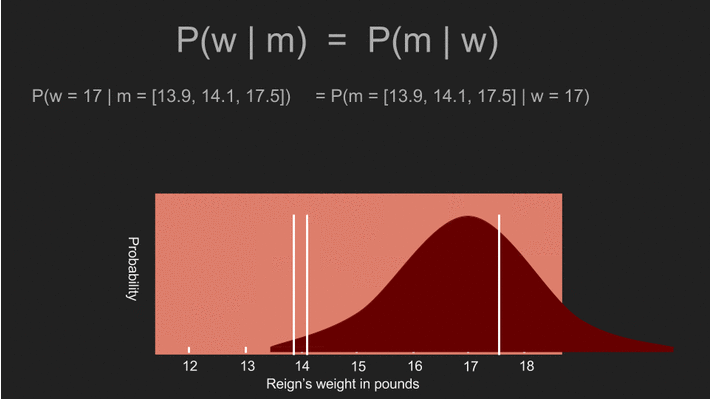At this point we can use every possible value of Reign’s weight and calculate the likelihood of getting our three measurements. For instance, our measurements would be extremely unlikely if Reign’s weight was one thousand pounds. However they would be quite likely if her weight was actually 14 pounds or 16 pounds. We can go through and, using every hypothetical value of her weight, calculate the likelihood of us getting the measurements that we got. This is P(m | w). Thanks to our uniform prior, this is also P(w | m), the posterior distribution.

It's not a matter of chance that this looks a lot like the answer we got by taking the mean, standard deviation and standard error. In fact the two are exactly the same. Using a uniform prior gives the traditional statistical estimate of the result. The location of the peak of this curve, the mean, at 15.2 pounds is also called the maximum likelihood estimate (MLE) of the weight.

Although we used Bayes’ Theorem, we’re still no closer to a useful estimate. To get this, we will need to make our prior non-uniform. A prior distribution represents our beliefs about something before we take any measurements. A uniform prior shows that we believe every possible outcome is equally likely. This is rarely the case. We often know something about the quantity we are measuring. Ages are always greater than zero. Temperatures are always greater than -276 Celsius. Adult heights are rarely greater than 8 feet. And sometimes we have additional domain knowledge that some values are more likely to occur in others.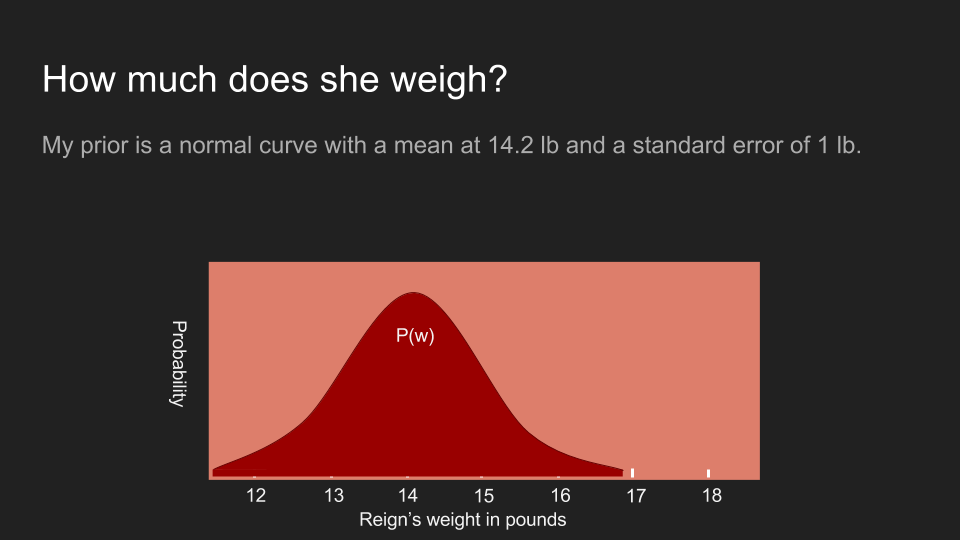In Reign’s case I do have additional information. I know that the last time I came to the vet she weighed in at 14.2 pounds. I also know that she doesn't feel noticeably heavier or lighter to me, although my arm is not a very sensitive scale. Because of this, I believe that she's about 14.2 pounds but might be a pound or two higher or lower. To represent this, I use a normal distribution with a peak at 14.2 pounds and with a standard deviation of a half pound.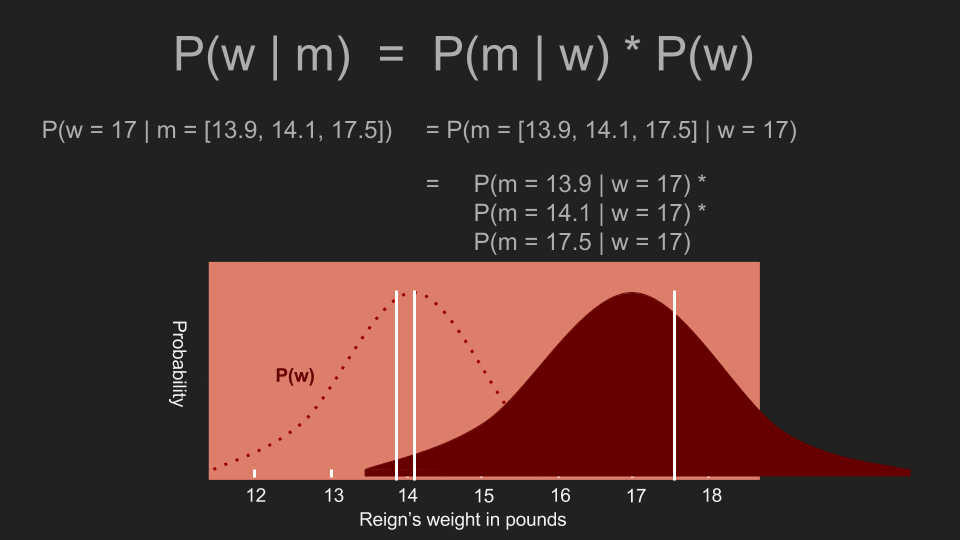With a prior in place, we can repeat the process of calculating our posterior. To do this, we consider the possibility that Reign’s weight is a certain value, say 17 pounds. Then we multiply the likelihood that she is actually 17 pounds (according to our prior) by the conditional probability of getting the measurements we did if she was 17 pounds. Then we repeat this for every other possible weight. The effect of the prior is to squash is down some probabilities and amplify others. In our case, it puts more weight on measurements in the 13-15 pound range, and much less weight on measurements outside it. This is in contrast to the uniform prior. It gave a decent possibility that Reign’s actual weight was 17 pounds. With the non-uniform prior, 17 pounds falls toward the tail of the distribution. Multiplying by that possibility drives the likelihood of a 17 pound weight down very low.By calculating the probability of each possible weight for Reign, we generate a new posterior. The peak of the posterior distribution is also known as the maximum a posteriori estimate or MAP, in our case 14.1 pounds. This is noticeably different than what we calculated before with a uniform prior. It's also a much narrower peak, which allows us to make a more confident estimate. Now we can see that Reign’s weight hasn’t changed much and her portion size can stay where it is.

By incorporating what we already knew about what we were measuring, we were able to make a more accurate estimate with more confidence than we would have been able to otherwise. It allowed us to make good use of a very small data set. Our prior assigned a very low probability to our 17.5 pound measurement. This is almost the same as rejecting the measurement as an outlier. But instead of doing outlier detection based on intuition and common sense, Bayes’ Theorem allows us to do it in with math.

As a side note, we assumed that the P(m) term was uniform, but if we happened to know that our scale was biased in some way, we could have reflected that in our P(m). If the scale only reported even numbers or returned a reading of “2.0” ten percent of the time, or generated random measurements every third try, we could have crafted P(m) to reflect this and it would have improved the accuracy of our posterior.

### Avoiding Bayesian traps

Weighing Reign showed the benefits of Bayesian inference, but there are also pitfalls. We improved our estimate by making some assumptions about the answer, but the whole purpose of measuring something is to learn about it. If we assume that we already know the answer then we may be censoring the data. Mark Twain put the danger of strong priors succinctly. “It ain't what you don't know that gets you into trouble. It's what you know for sure that just ain't so.”

If we were to start with a strong prior assumption that Reign’s weight is between 13 and 15 pounds, then we would never be able to detect if her weight had actually fallen to 12.5. Our prior would assign zero probability to that outcome, and every measurement we got below 13 pounds would be disregarded, no matter how many times we measured.

Luckily, there is a way to hedge our bets and avoid blindly eliminating possibilities. That is to assign at least a small probability to every outcome. That way, if by some quirk of physics Reign actually did weigh 1,000 pounds, the measurements that we gathered would be able to reflect that in the posterior. This is one reason that normal distributions are commonly used as priors. They concentrate most of our belief around a small range of outcomes, but have very long tails that never become entirely zero no matter how far they stretch.In this, the Red Queen provides a good role model:

Alice laughed: "There's no use trying," she said; "one can't believe impossible things."
"I daresay you haven't had much practice," said the Queen. "When I was younger, I always did it for half an hour a day. Why, sometimes I've believed as many as six impossible things before breakfast."
- Lewis Carroll (Alice’s Adventures in Wonderland)

Corrections: Thank you to those who have spotted typos and errors! I owe each of you a beverage of your choice: Justin Fortier and Irina Max.

Original. Reposted with permission.

Related:

Top Stories Past 30 Days
Most SharedGet KDnuggets, a leading newsletter on AI, Data Science, and Machine Learning